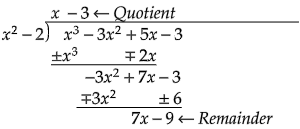# divide the polynomial $p( x)$ by the polynomial $g( x)$ and find the quotient and remainder in each of the following: $( p(x)=x^{3}-3 x^{2}+5 x-3$, $g(x)=x^{2}-2$.

Given: $( p(x)=x^{3}-3 x^{2}+5 x-3$, $g(x)=x^{2}-2$.

To do: To divide the polynomial $p( x)$ by the polynomial $g( x)$ and find the quotient and remainder.

Solution:

As given, $( p(x)=x^{3}-3 x^{2}+5 x-3$, $g(x)=x^{2}-2$

On Dividing $p( x)$ by $g( x)$ by long division:Quotient$=x-3$

Remainder$=7x-9$.